Field Theory - Online Tutorial 3

by Kevin Tang , last update: Jul 1st, 2014

[main pages]

Q1 -

Given a spherical capacitor that have a inner and outer radius a [m] and b [m].

Where voltage will be following... V(r=a) = 5 [V], V(r=b) = 0[V] as shown in Figure #1.  Given ε = εo and conductivity constant = σ

Find the following...

1a) Write down Laplace's Equation

1b) V (a < r < b)

1c) E

1d) ρsa when r = a

1e) Q when r= a

1f) C at r =a

1g) Energy Density

1h) Total Energy

1i) G conductivity, when r = a, given conductivity constant = σ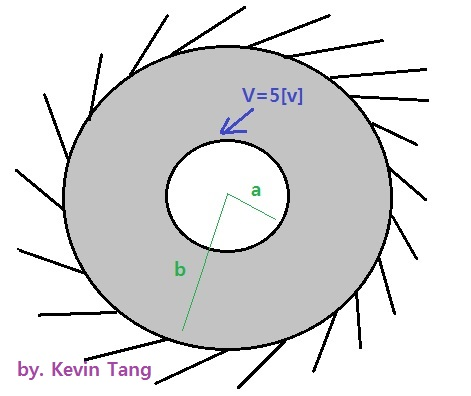Figure # 1

1a) Write down Laplace's Equation

Solving Time : 10 sec

If you are not sure which one is Laplace's Equation, please review it right now~

 A ∇V = 0 Wrong Answer B ∇E = 0 Wrong Answer C ∇2V = 0 Correct! D ∇2E = 0 Wrong Answer

.

.

.

.

.

.

.

SOLUTION 1a)

Yes, very simple ... its 2V = 0

Notice, Laplace's equation is from the Poisson's equation, its a "special case" of Poisson equation, where as following...

2V = − ρv/ ε  .... Poisson's equation

when you are in charge-free region, which ρv = 0

so it became.. 2V = 0

1b) Find V (a<r<b)

Solving Time: 10 min   ... lets give a try :D

V (a < r < b) = ? which one?

 A (5/(1/b − 1/a))(1/b −1/r) [V] Correct! B (5/(1/a − 1/b)(1/a − 1/r)[V] Wrong Answer C (1/(1/b − 1/a))(1/a − 1/r)[V] Wrong Answer

SOLUTION 1b)

Here is how you solve it, please actually go over it at least "once" by yourself.(Please don't forget the condition, that r CANNOT equal to zero)

Next, our target is to find A and B, 2 unknown so you need at least 2 equations,

sub the voltage value, V(r=a) = 5 and V(r= b) = 0 to make 2 equations.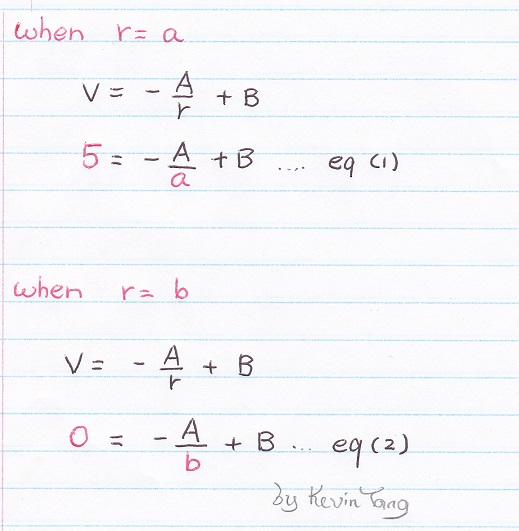Now, let's find out A = ? and  B = ?...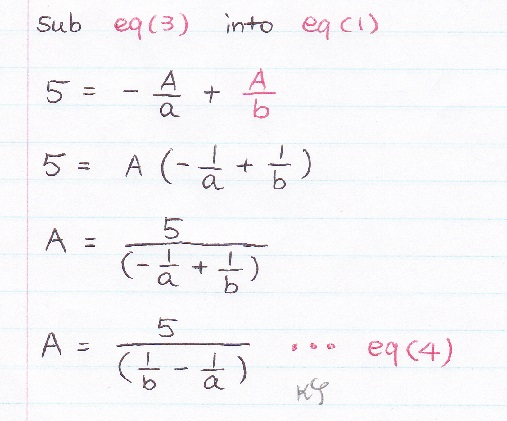Almost finished.......

1c) Find E

Maximum solving time: 5 min

 A −1/r2 (5/(1/a − 1/b)) ar [V/m] Wrong Answer B 1/r2 (5/(1/a − 1/b)) ar [V/m] Correct! C 1/a2 (5/(1/a − 1/b)) ar [V/m] Wrong Answer

SOLUTION 1c)

Simply E = −V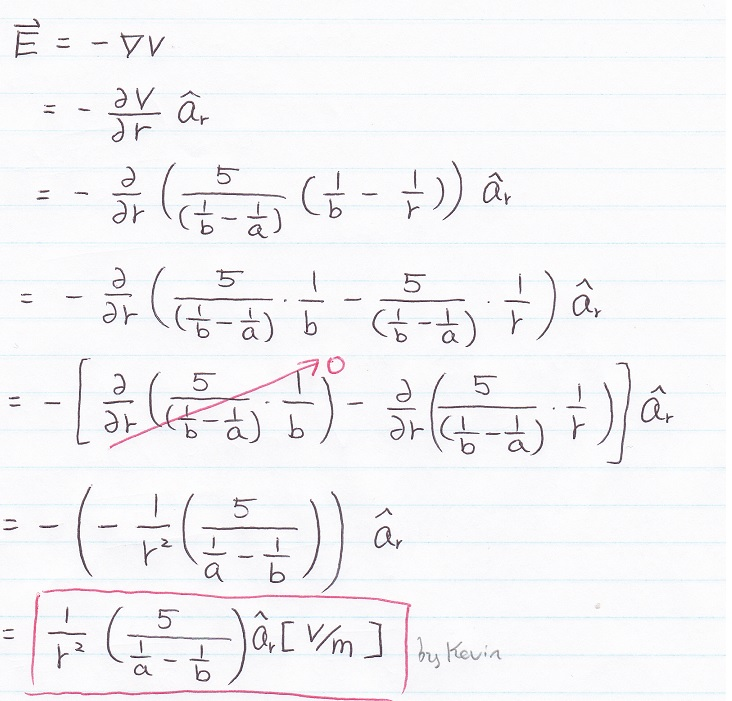1d) Find ρsa at r = a  (where ε = εo)

Suggest Solving Time: 5 min

 A εo/a2 (5/(1/a − 1/b)) ar [C/m2] Wrong Answer B εo/r2 (5/(1/a − 1/b)) [C/m2] Wrong Answer C εo/a2 (5/(1/a − 1/b)) [C/m2] Correct!

.

.

.

.

.

.

SOLUTION 1d)

* If you choice A ... ρsa is a scalar not a vector.

* If you choice B ... don't forget to sub r = a at the end.

* If you choice C ... you are cool :D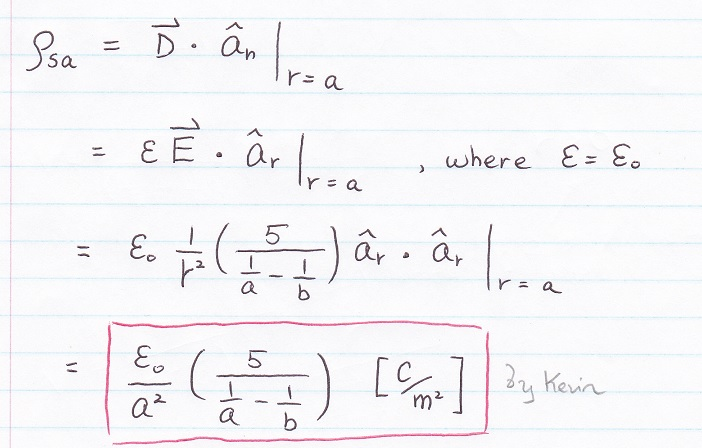1e) Find Q when r= a

Suggest Solving Time: 2 min

SOLUTION 1e)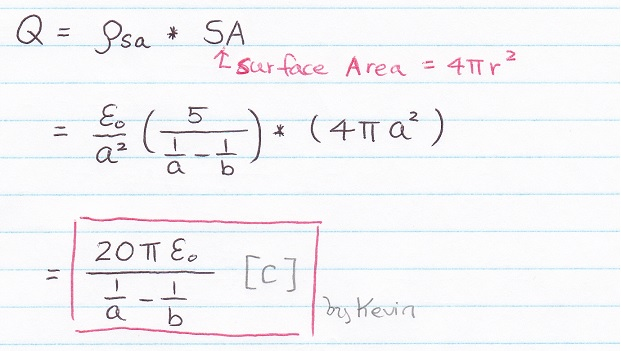1f) Find C when r = a

Suggest Solving Time: 1 min

SOLUTION 1f)1g) Find Energy Density

Suggest Solving Time: 2 min

SOLUTION 1g)

The symbol of energy density is small case we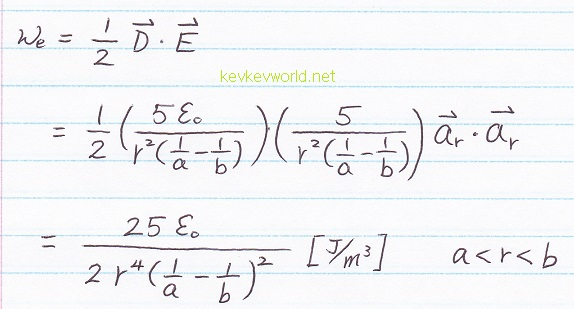1h) Find Total Energy

Suggest Solving Time: 3 min

SOLUTION 1h)

The symbol of Total Energy is capital case We* Note: The integral of surface area respect to the change of radius, can lead you directly to the volume, no need to perform triple integral here.1i) G conductivity, when r = a, given conductivity constant = σ

Suggest Solving Time: 3 min

SOLUTION 1i)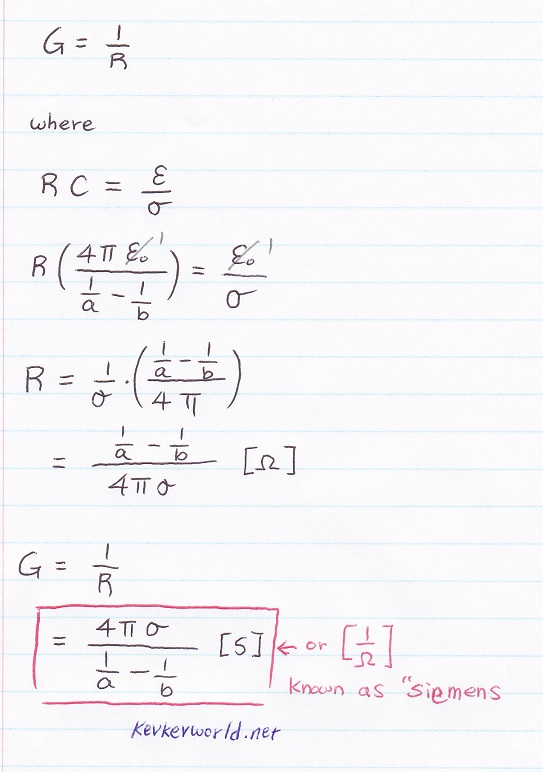Q2

An infinitely long transmission line consisting of two concentric cylinders having their axes along z-axis.  The inner conductor has radius "a"

and carrying current I, while the outer conductor has inner radius b and thickness t and carries return current - I.

Find the following...

a) Draw the diagram (show the detail label)

b) Find the magnetic field strength H everywhere

c) Find the magnetic flux B everywhere

d) Find the self inductance of inner conductor

SOLUTION a)

 A a)Wrong Answer B b)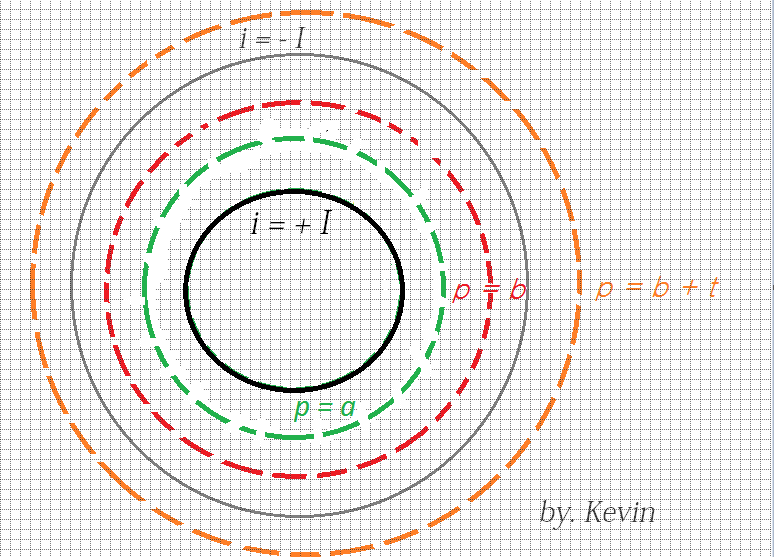Correct Answer

If you choice A, you got it wrong, as it said the inner conductor has radius of "a", the current is running inside it, so the i = +I will be inside "a"

If you choice B, you are cool~ :D

b) Find the magnetic field strength H everywhere

SOLUTION b)

First you should able to see there are "4" regions.

Region 1 :  0 < ρ < a

Region 2 :  a < ρ < b

Region 3 :  b < ρ < b + t

Region 4 :  b+ t < ρ

Remember, left side stay the same, only the right hand side is chaging

For Region 1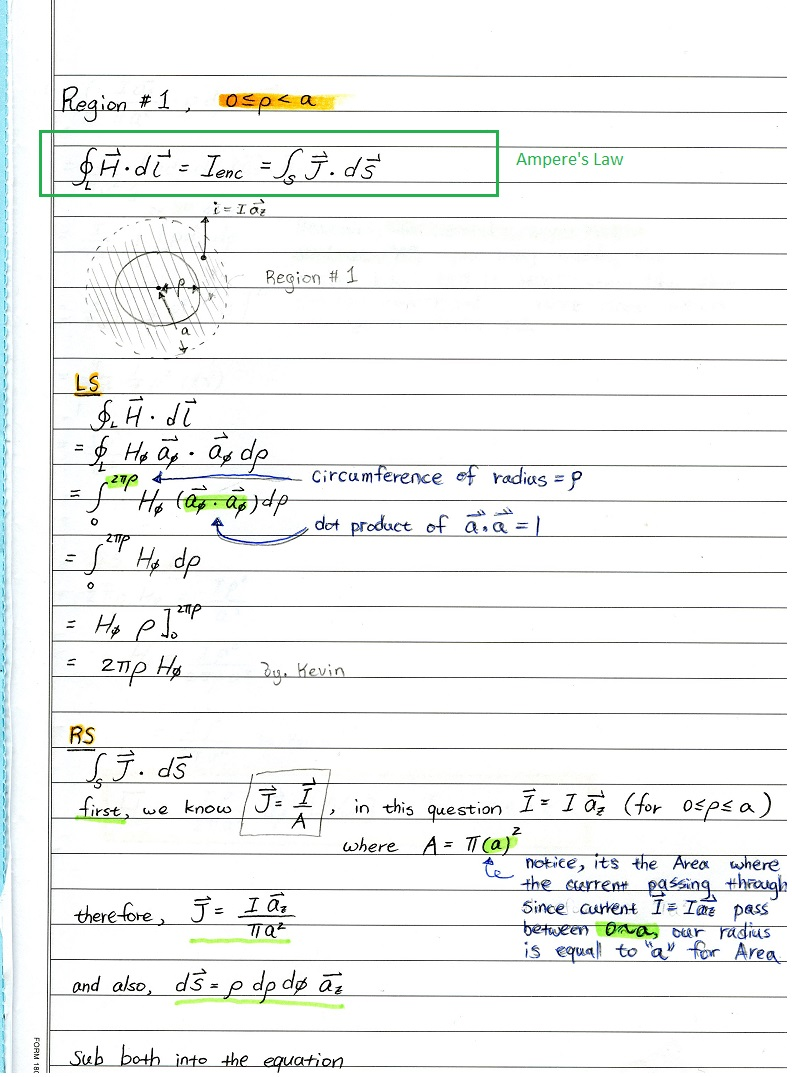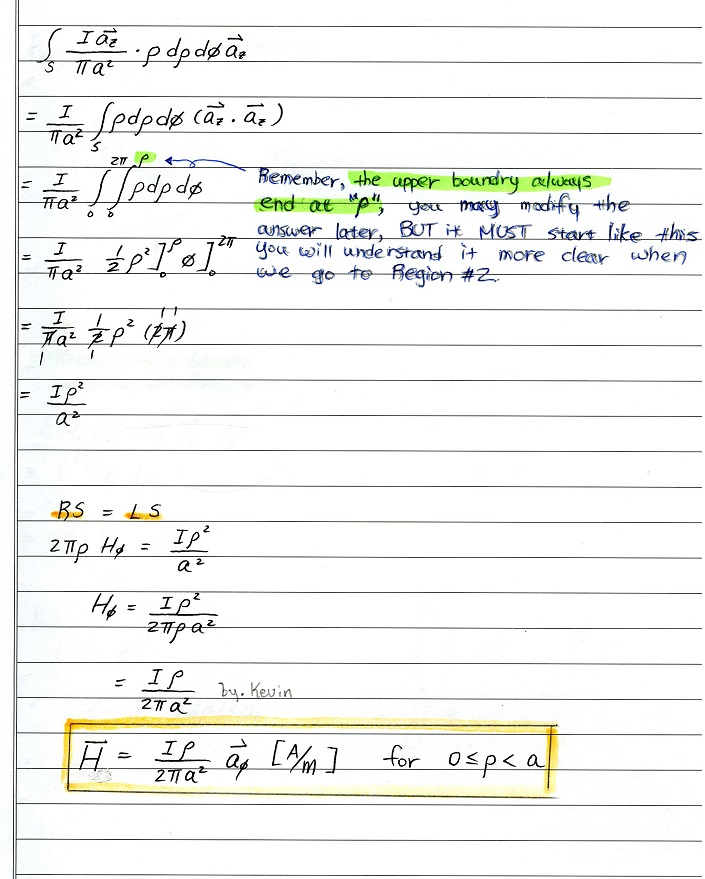For Region 2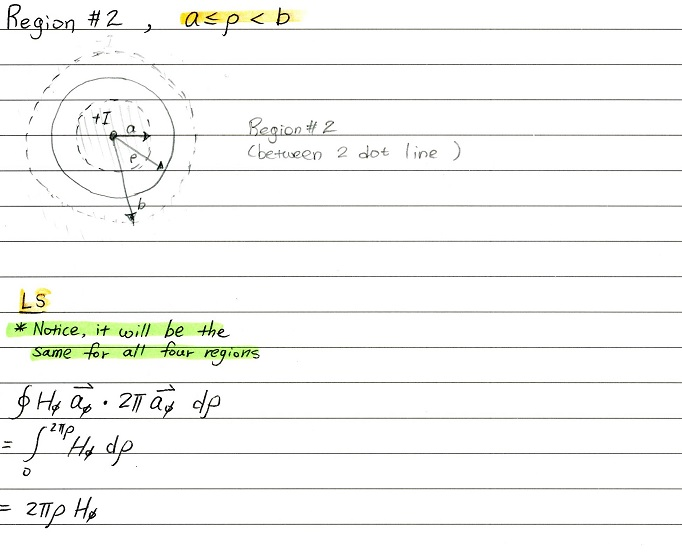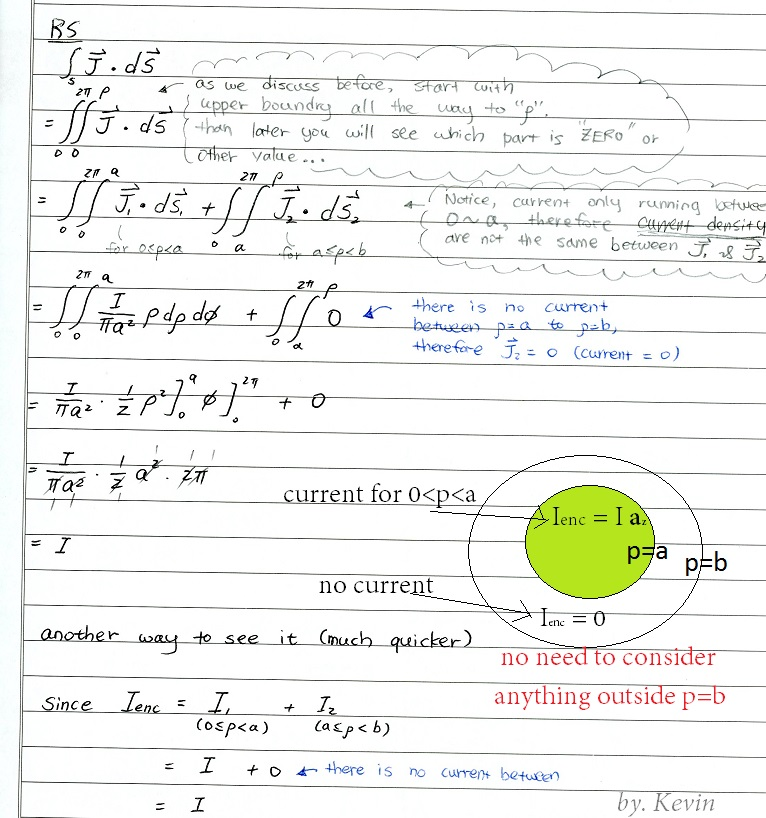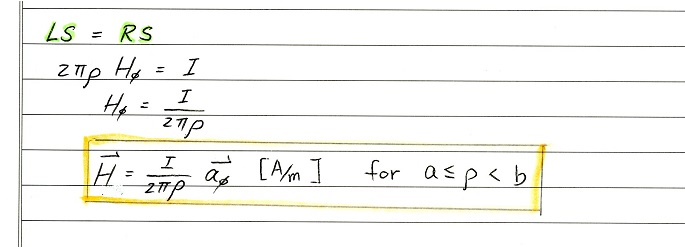For Region 3
Notice, the LS side is still the same (line integral part)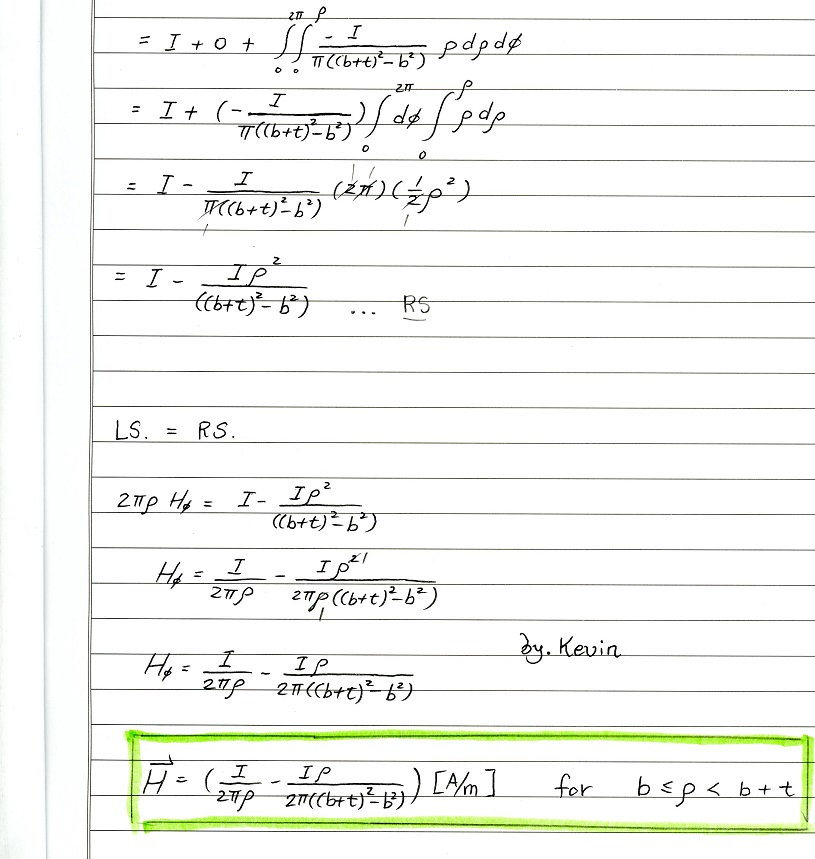For Region 4
H = 0 [A/m].... why?

Due to the the total enclosed current equal to zero when you are outside b+t, as I + (-I) = 0

c) Find

d) Self Inductance

Last note:

Good luck, its my very pleasure to work with all of you :)

You may leave me any message if you have any questions, I will try to answer ASAP, thanks and good luck :)